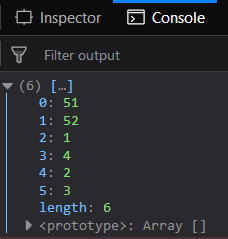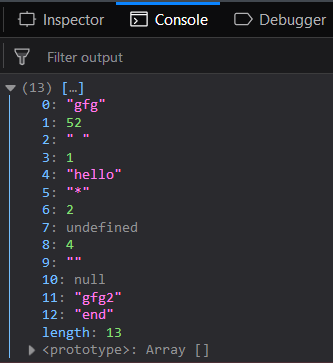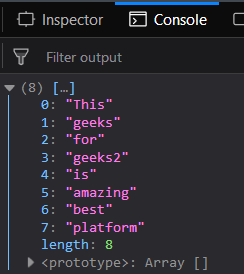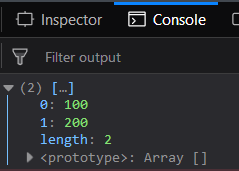Open in App
Not now

# Underscore.js _.union() Function

• Last Updated : 24 Nov, 2021

The Underscore.js is a JavaScript library that provides a lot of useful functions like the map, filter, invoke etc even without using any built-in objects.
The _.union() function is used to take n number of arrays and return a new array with the unique terms in all those arrays (union of all array). In the new array the order of the elements is same like it is mentioned in all the passed arrays. The first occurrence of each array is only included in the resultant array.

Syntax:

`_.union( *arrays )`

Parameters: This function accepts single parameter arrays which is the collection of multiple array list. The array list are separated by , operator.

Return value: It returns an array which contains unique elements of all the elements in n passed arrays.

Passing a list of numbers to _.union() function: The ._union() function takes the element from the list one by one checks whether it is already present in the resultant array or not. If it is present then it just ignores it otherwise adds it to the resultant array. The final result contains the union of array.

Example:

 ```<``html``>``    ``<``head``>``        ``<``script` `src` `= ``"https://cdnjs.cloudflare.com/ajax/libs/underscore.js/1.9.1/underscore-min.js"` `>``        ````    ````    ``<``body``>``        ``<``script` `type``=``"text/javascript"``>``            ``console.log(_.union([51, 52, 1, 4], ``                                ``[1, 2, 3, 4], ``                                ``[1, 2]));``        ````    ````                    `

Output:Passing a combination of words, false values and numbers to the _.union() function: Passing any kind of elements whether it is a number, word or even false elements like empty strings, null values etc, the _.union() function will not distinguish between them. It will rather treat every element in the same manner. The further process will be the same.

Example:

 ```<``html``>``    ``<``head``>``        ``<``script` `src` `= ``"https://cdnjs.cloudflare.com/ajax/libs/underscore.js/1.9.1/underscore-min.js"` `>``        ````    ````    ``<``body``>``        ``<``script` `type``=``"text/javascript"``>``            ``console.log(_.union(["gfg", 52, " ", 1, "hello"], ``                                ``['*', 2, undefined, 4], ``                                ``['', null], ``                                ``["gfg2", "end"]));``        ````    ````                    `

Output:Passing a set of strings to the _.union() function: Pass a set of strings to this function so as to get the common of all the n arrays passed in the result. The processing will occur in the same way. Only the words given in the second parameter will be excluded.

Example:

 ```<``html``>``    ``<``head``>``        ``<``script` `src` `= ``"https://cdnjs.cloudflare.com/ajax/libs/underscore.js/1.9.1/underscore-min.js"` `>``        ````    ````    ``<``body``>``        ``<``script` `type``=``"text/javascript"``>``            ``console.log(_.union(["This", "geeks"], ``                                ``['for', "geeks2", "is", "amazing"],``                                ``["This", "is", "best", "platform"]));``        ````    ````                    `

Output:Passing arrays with same elements to the _.union() function: If pass arrays to the _.union() function and they have the same elements then union of all the arrays will be the first array itself. All the elements will be common and hence will be present in the result given after union.

Example:

 ```<``html``>``    ``<``head``>``        ``<``script` `src` `= ``"https://cdnjs.cloudflare.com/ajax/libs/underscore.js/1.9.1/underscore-min.js"` `>``        ````    ````    ``<``body``>``        ``<``script` `type``=``"text/javascript"``>``            ``console.log(_.union([100, 200], ``                                ``[100, 200], ``                                ``[100, 200], ``                                ``[100, 200], ``                                ``[100, 200], ``                                ``[100, 200]));``        ````    ````                    `

Output:Note: These commands will not work in Google console or in Firefox as for these additional files need to be added which they didn’t have added. So, add the given links to your HTML file and then run them.

 `<``script` `type``=``"text/javascript"` `src` `= ``"https://cdnjs.cloudflare.com/ajax/libs/underscore.js/1.9.1/underscore-min.js"``> `` `

My Personal Notes arrow_drop_up